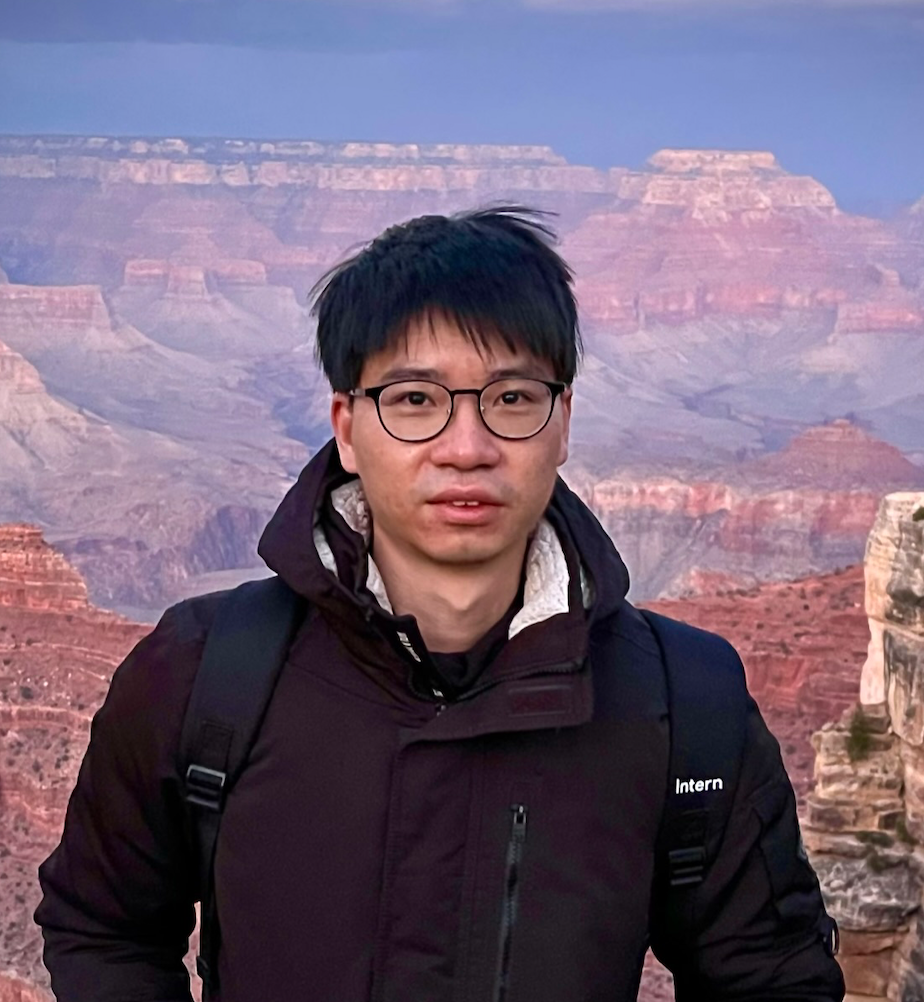Timezone: »

Privacy Amplification via Compression: Achieving the Optimal Privacy-Accuracy-Communication Trade-off in Distributed Mean Estimation
Wei-Ning Chen · Dan Song · Ayfer Ozgur · Peter Kairouz

Privacy and communication constraints are two major bottlenecks in federated learning (FL) and analytics (FA). We study the optimal accuracy of mean and frequency estimation for FL and FA respectively under joint communication and $(\varepsilon, \delta)$-differential privacy (DP) constraints. We consider both the central and the multi-message shuffling DP models. We show that in order to achieve the optimal $\ell_2$ error under $(\varepsilon, \delta)$-DP, it is sufficient for each client to send $\Theta\left( n \min\left(\varepsilon, \varepsilon^2\right)\right)$ bits for FL and $\Theta\left(\log\left( n\min\left(\varepsilon, \varepsilon^2\right) \right)\right)$ bits for FA to the server, where $n$ is the number of clients. We propose two different ways to leverage compression for privacy amplification and achieve the optimal privacy-communication-accuracy trade-off. In both cases, each client communicates only partial information about its sample and we show that privacy is amplified by randomly selecting the part contributed by each client. In the first method, the random selection is revealed to the server, which results in a central DP guarantee with optimal privacy-communication-accuracy trade-off. In the second method, the random data parts at each client are privatized locally and anonymized by a secure shuffler, eliminating the need for a trusted server. This results in a multi-message shuffling scheme with the same optimal trade-off. As a result, our paper establishes the optimal three-way trade-off between privacy, communication, and accuracy for both the central DP and multi-message shuffling frameworks.

#### Author Information

##### Wei-Ning Chen (Stanford University)Wei-Ning Chen is currently a Ph.D. student at Stanford EE under the support of Stanford Graduate Fellowship (SGF). His research interests broadly lie in information-theoretic and algorithmic aspects of data science. He adopt tools mainly from information theory, theoretical machine learning, and statistical inference, with a current focus on distributed inference, federated learning and differential privacy.